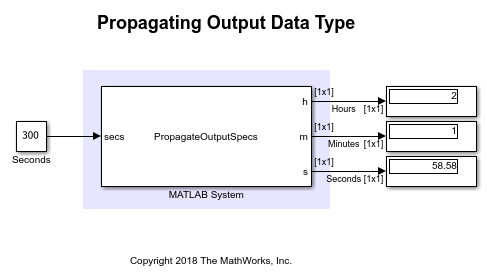# Specify Output Characteristics of MATLAB System Block

This example shows how to specify output size, data type and complexity of a MATLAB System block.

### System objects

System objects allow you to implement algorithms using MATLAB. System objects are a specialized kind of MATLAB object, designed specifically for implementing and simulating dynamic systems with inputs that change over time.

After you define a System object, you can include it in a Simulink model using a MATLAB System block.

### Model Description

This example has a MATLAB System block that adds input number of seconds to the current time and produces the resulting hours, minutes and seconds as outputs. The output values from each output port are displayed in the Display blocks.This example, shows how to specify output size, data type and complexity of a System object. You specify the output properties when MATLAB System block cannot infer them automatically. The MATLAB System block uses the System object `PropagateOutputSpecs` that implements methods to propagate input data type, size and complexity.

### System Object Class Definition

You can access MATLAB source code used by the MATLAB System block by clicking the "Source code" hyperlink from the block dialog. The System object `PropagateOutputSpecs` implements the `stepImpl` method that adds the input value in seconds to current time and outputs the resulting hours, minutes and seconds. The `stepImpl` method uses `datetime` function to calculate its output. Since `datetime` function is not supported for code generation, MATLAB System block cannot infer the output specifications automatically. The System object implements the following methods to specify output properties:

• `getOutputSizeImpl` - Specify output size

• `getOutputDataTypeImpl` - Specify output data type

• `isOutputComplexImpl` - Specify output complexity

• `isOutputFixedSizeImpl` - Specify whether output can be variable-size

```classdef PropagateOutputSpecs < matlab.System % PropagateOutputSpecs Propagation in Simulink methods(Access = protected) function [h, m, s] = stepImpl(~, secs) % Add input hours, minutes and seconds to current time d = datetime; d = d + seconds(secs); h = hour(d); m = minute(d); s = second(d); end function [o1, o2, o3] = getOutputSizeImpl(obj) % Return size for output port to be same as input port inSize = propagatedInputSize(obj, 1); o1 = inSize; o2 = inSize; o3 = inSize; end function [o1, o2, o3] = getOutputDataTypeImpl(obj) % Return data type for output port to be same as input port inType = propagatedInputDataType(obj, 1); o1 = inType; o2 = inType; o3 = inType; end function [o1, o2, o3] = isOutputComplexImpl(~) % Return output port complexity to be real o1 = false; o2 = false; o3 = false; end function [o1, o2, o3] = isOutputFixedSizeImpl(~) % Return true for each output port with fixed size o1 = true; o2 = true; o3 = true; end end end ```